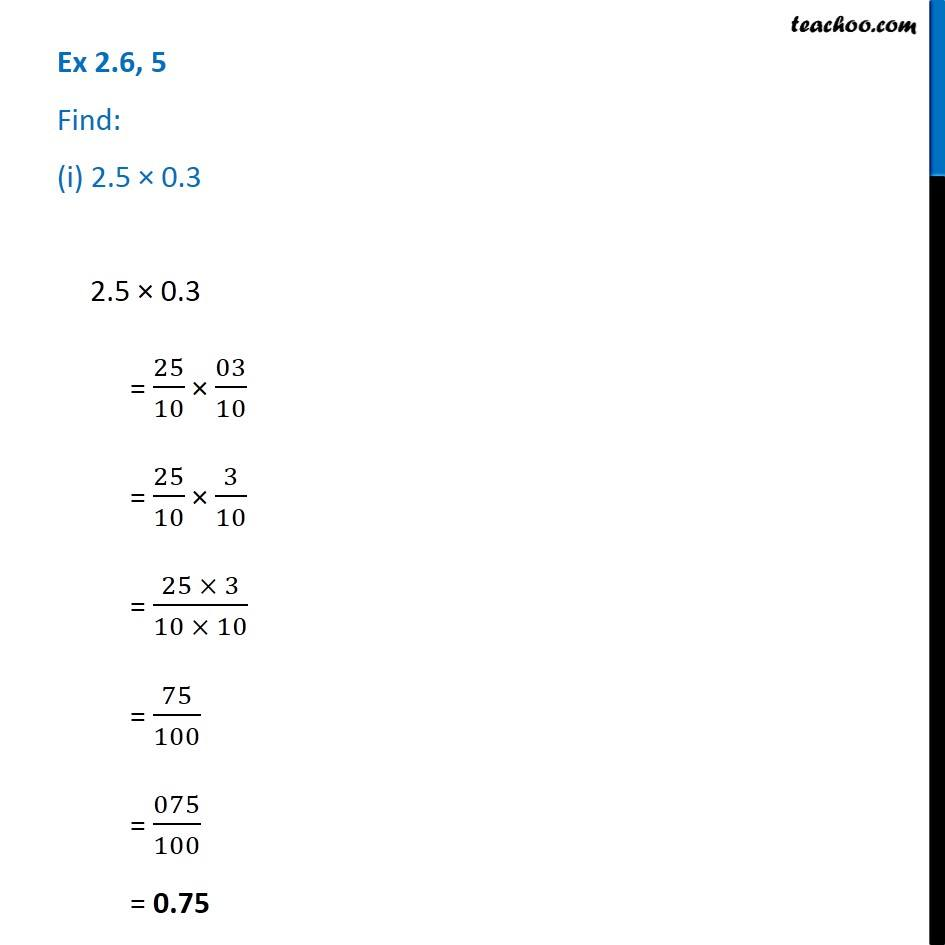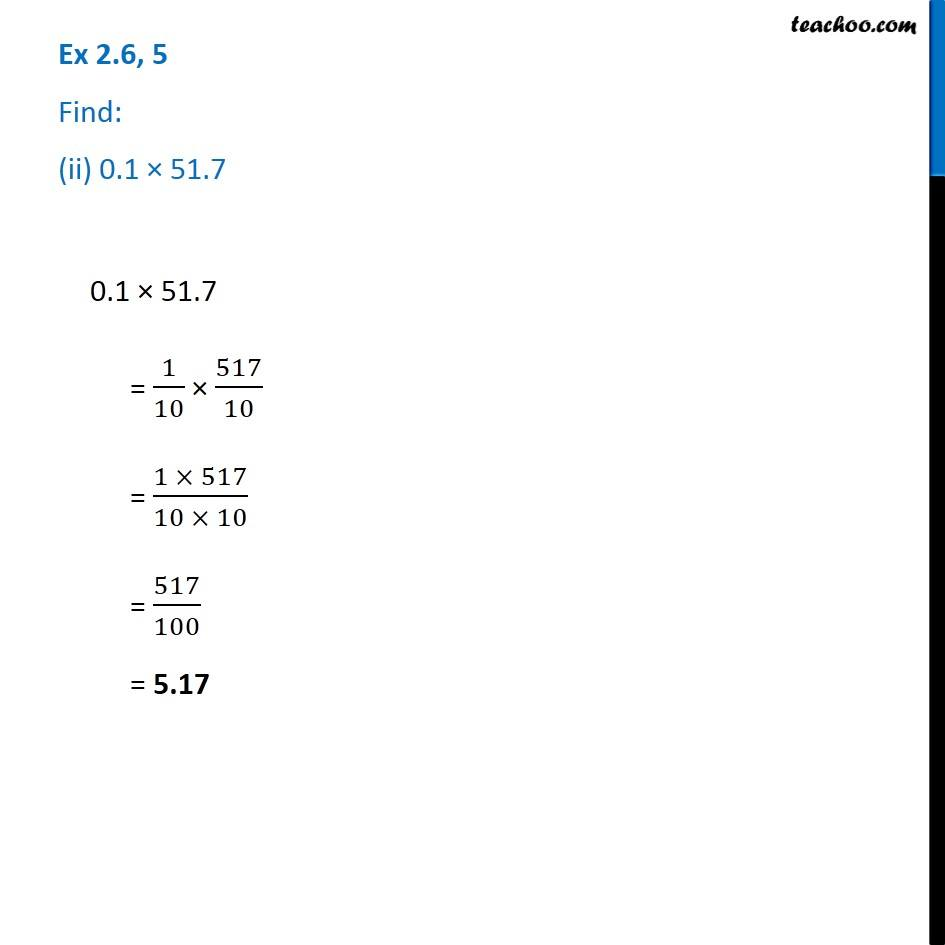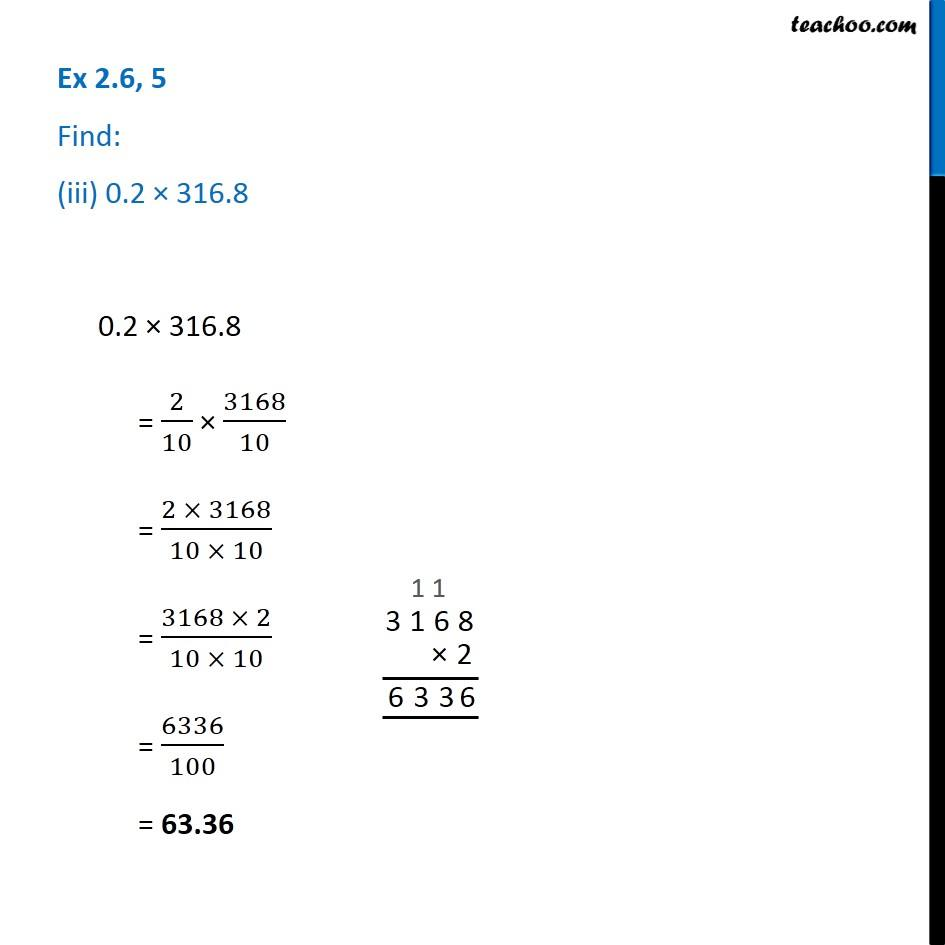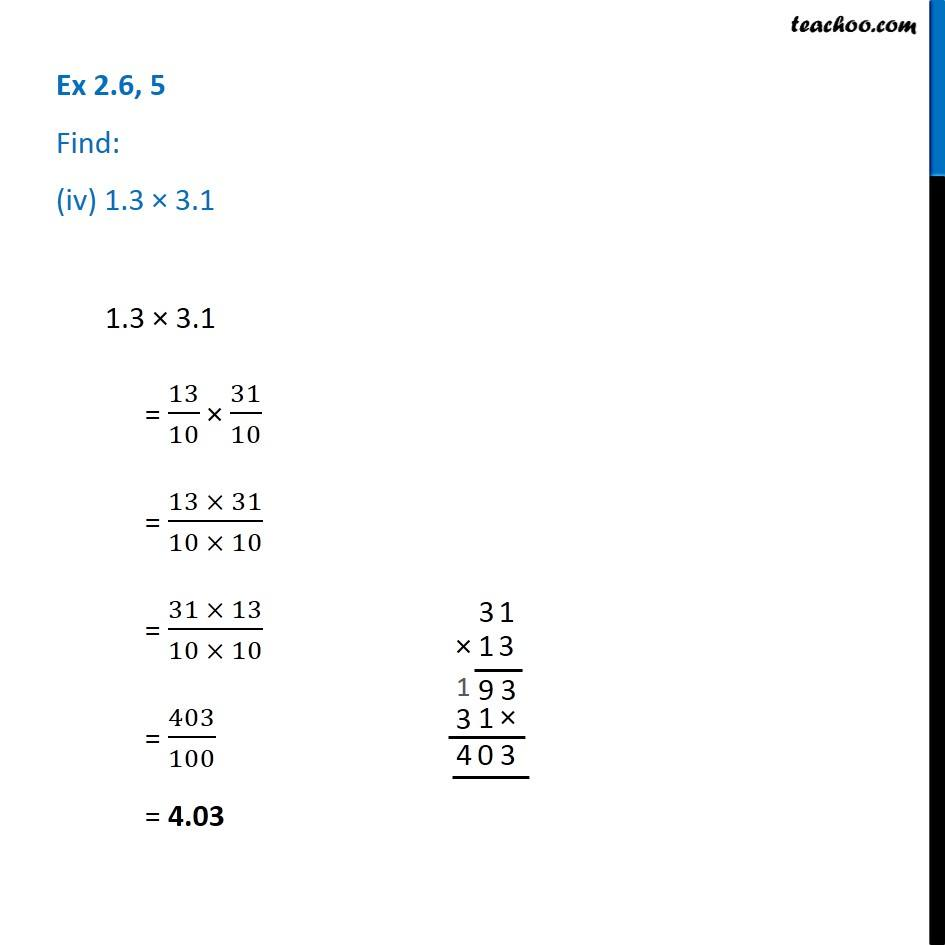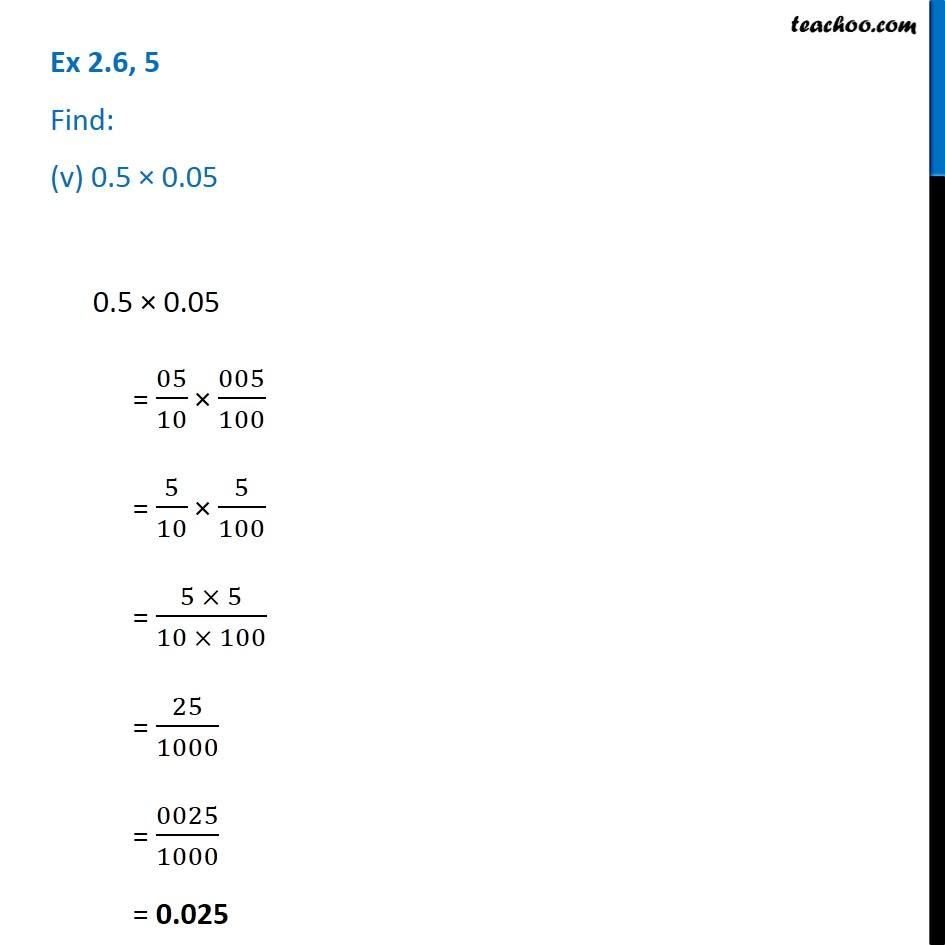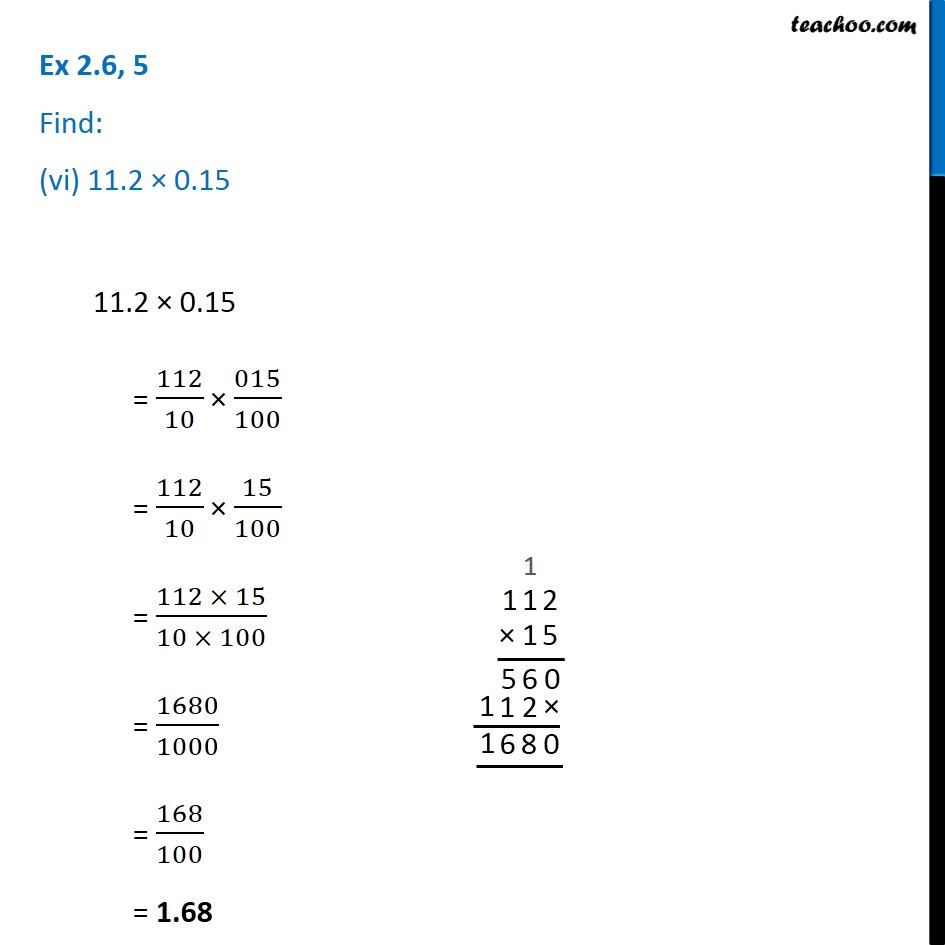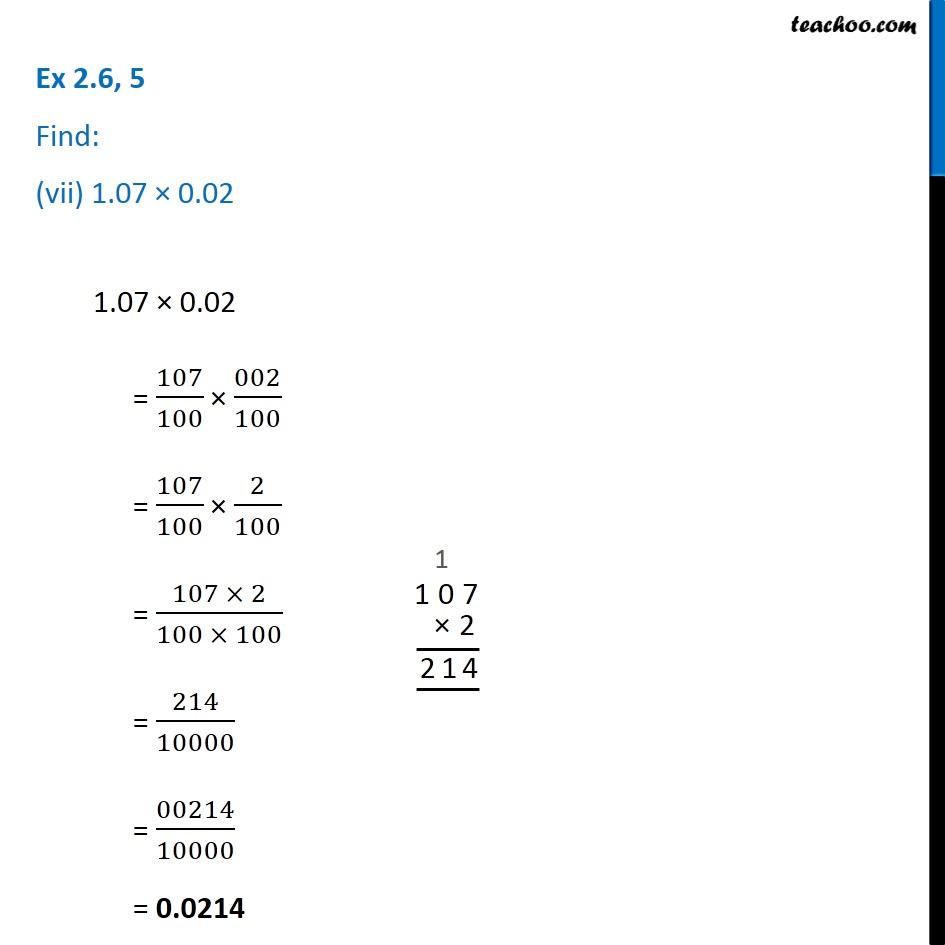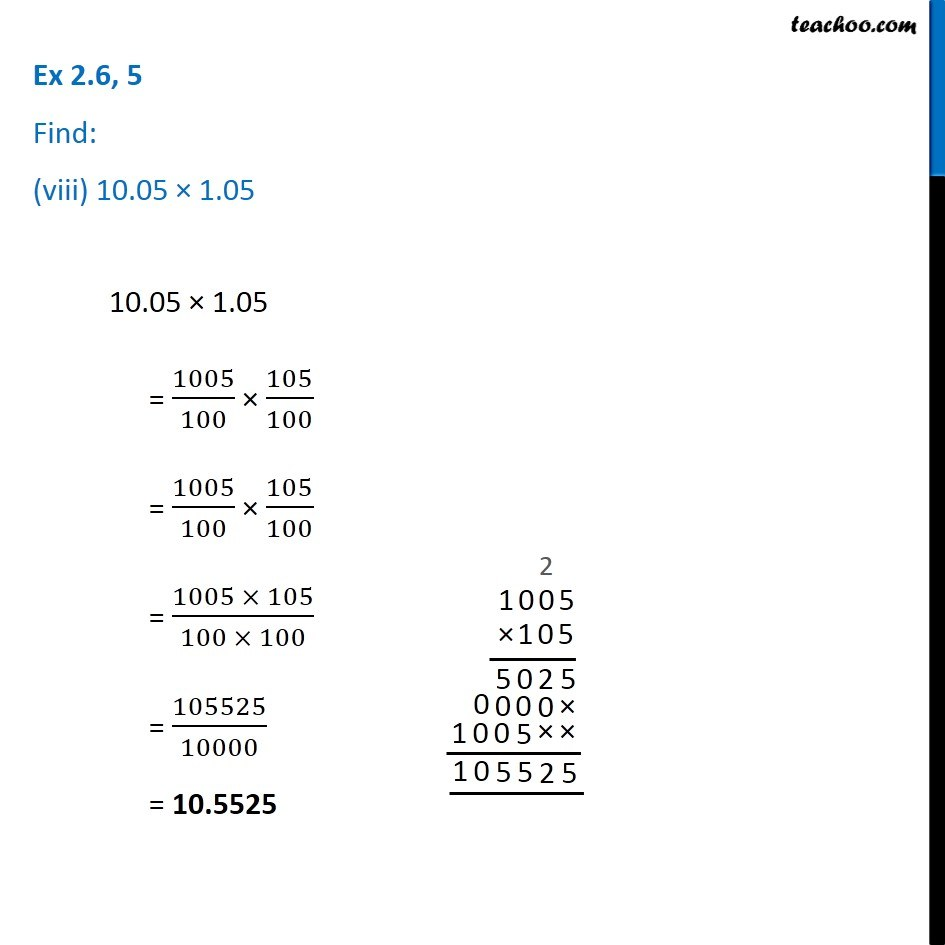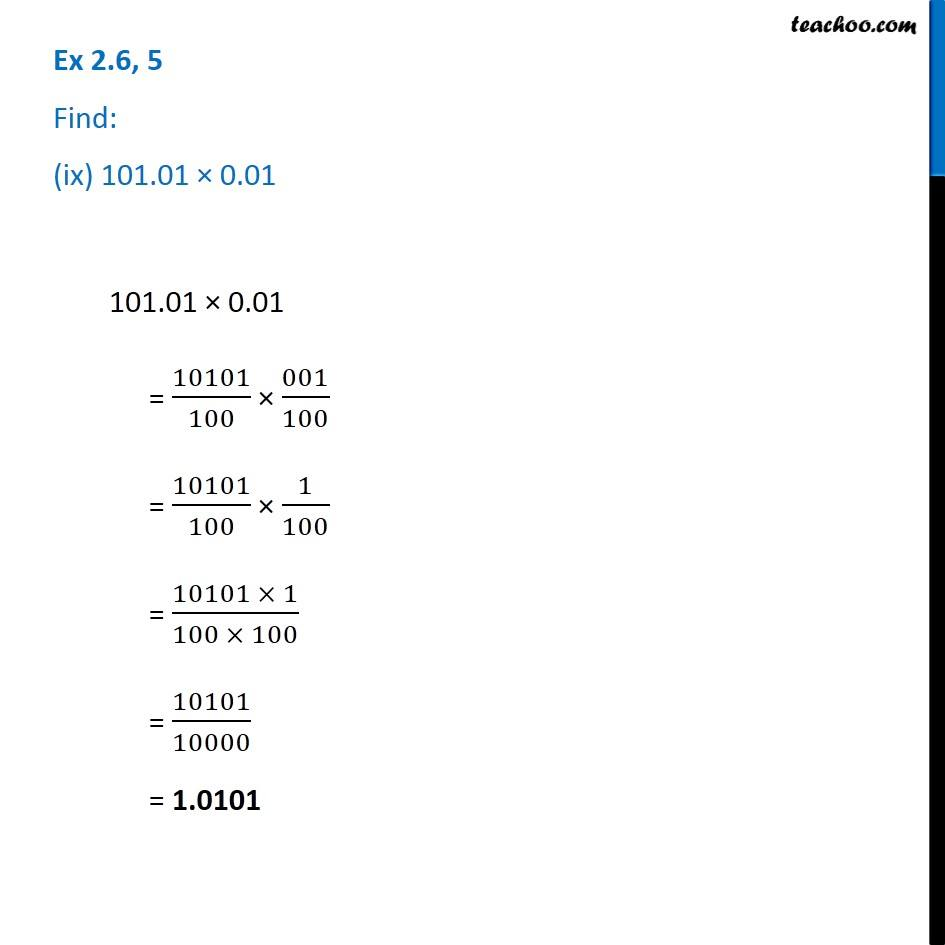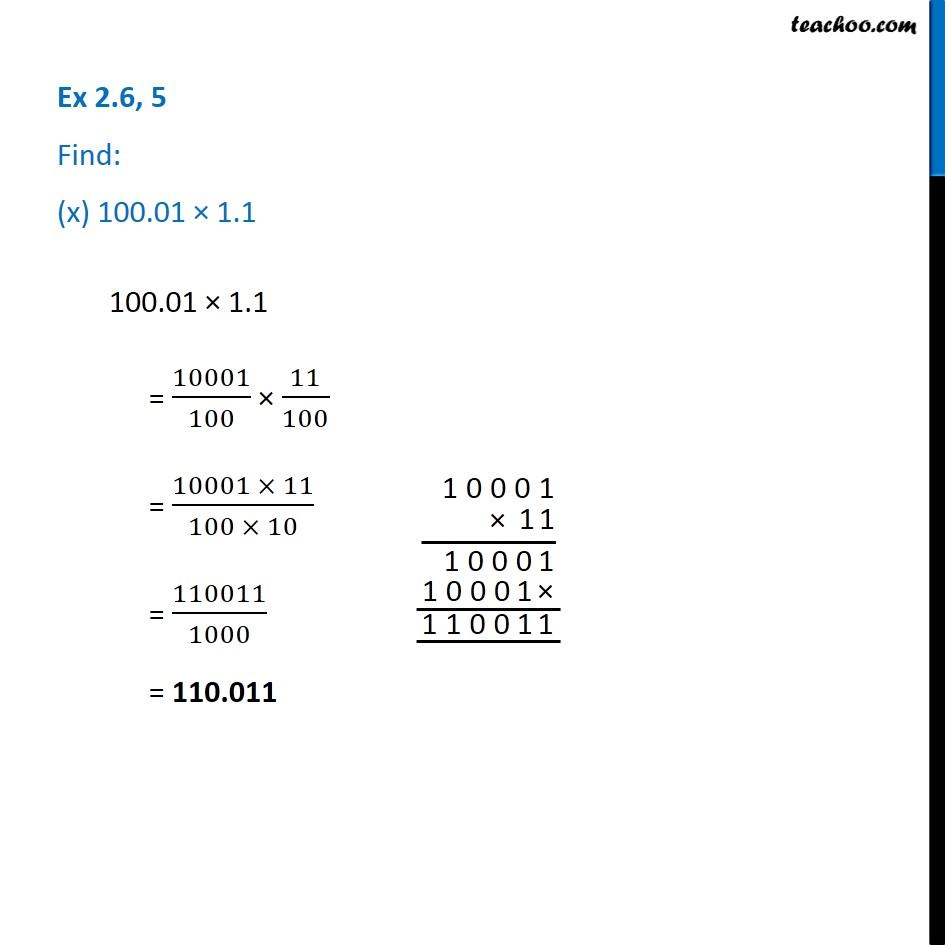1. Chapter 2 Class 7 Fractions and Decimals
2. Concept wise
3. Multiplication of Decimals

Transcript

Ex 2.6, 5 Find: (i) 2.5 × 0.32.5 × 0.3 = 25/10 × 03/10 = 25/10 × 3/10 = (25 × 3)/(10 × 10) = 75/100 = 075/100 = 0.75 Ex 2.6, 5 Find: (ii) 0.1 × 51.70.1 × 51.7 = 1/10 × 517/10 = (1 × 517)/(10 × 10) = 517/100 = 5.17 Ex 2.6, 5 Find: (iii) 0.2 × 316.80.2 × 316.8 = 2/10 × 3168/10 = (2 × 3168)/(10 × 10) = (3168 × 2)/(10 × 10) = 6336/100 = 63.36 Ex 2.6, 5 Find: (iv) 1.3 × 3.11.3 × 3.1 = 13/10 × 31/10 = (13 × 31)/(10 × 10) = (31 × 13)/(10 × 10) = 403/100 = 4.03 Ex 2.6, 5 Find: (v) 0.5 × 0.050.5 × 0.05 = 05/10 × 005/100 = 5/10 × 5/100 = (5 × 5)/(10 × 100) = 25/1000 = 0025/1000 = 0.025 Ex 2.6, 5 Find: (vi) 11.2 × 0.15Ex 2.6, 5 Find: (vi) 11.2 × 0.15Ex 2.6, 5 Find: (vii) 1.07 × 0.021.07 × 0.02 = 107/100 × 002/100 = 107/100 × 2/100 = (107 × 2)/(100 × 100) = 214/10000 = 00214/10000 = 0.0214 Ex 2.6, 5 Find: (viii) 10.05 × 1.05 10.05 × 1.05 = 1005/100 × 105/100 = 1005/100 × 105/100 = (1005 × 105)/(100 × 100) = 105525/10000 = 10.5525 Ex 2.6, 5 Find: (ix) 101.01 × 0.01101.01 × 0.01 = 10101/100 × 001/100 = 10101/100 × 1/100 = (10101 × 1)/(100 × 100) = 10101/10000 = 1.0101 Ex 2.6, 5 Find: (x) 100.01 × 1.1100.01 × 1.1 = 10001/100 × 11/100 = (10001 × 11)/(100 × 10) = 110011/1000 = 110.011

Multiplication of Decimals